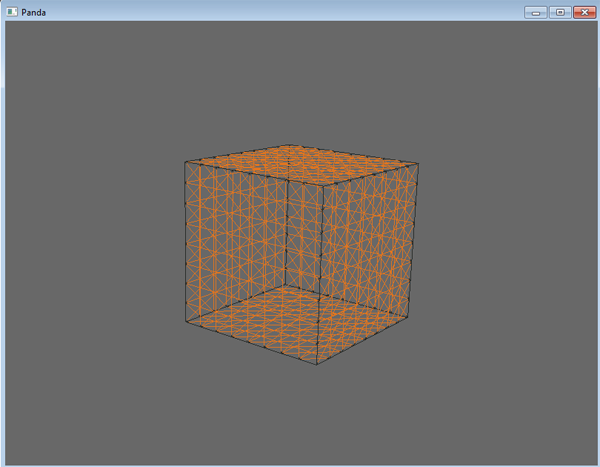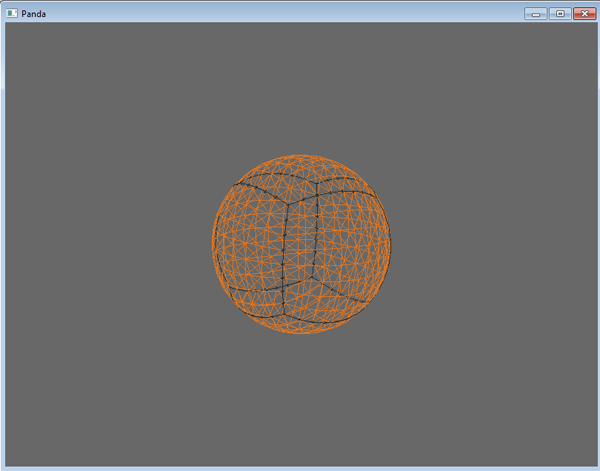Cube to sphere

Technology applied to the planets, asteroids

Before:After:Code:

planet.7z (11.1 KB)

F3 - Mode Wireframe

Thank you for this - I’m quite interested in procedural model deformation techniques!

However, I would like to point out that it can be done faster & simpler by just normalizing the vertex position vectors:

# -*- coding: utf-8 -*-
from direct.showbase.ShowBase import ShowBase
from pandac.PandaModules import *
from math import sqrt

class MyApp(ShowBase):

def __init__(self):

ShowBase.__init__(self)

planet.reparentTo(render)

geomNodeCollection = planet.findAllMatches('**/+GeomNode')

for nodePath in geomNodeCollection:

geomNode = nodePath.node()

for i in range(geomNode.getNumGeoms()):

geom = geomNode.modifyGeom(i)

vdata = geom.modifyVertexData()

vertex = GeomVertexRewriter(vdata, 'vertex')

while not vertex.isAtEnd():

v = Vec3(vertex.getData3f())
v.normalize()
vertex.setData3f(v)

self.accept("f3", self.toggleWireframe)

app = MyApp()
app.run()

Another advantage of the above code is that it works with any model, while your code will crash with a “math domain error” ValueError when a vertex lies too far from the model origin.

It’s also possible to “spherify” a model to a certain degree (instead of making it an exact unit-radius sphere) by slightly modifying the new code:

# -*- coding: utf-8 -*-
from direct.showbase.ShowBase import ShowBase
from pandac.PandaModules import *
from math import sqrt

class MyApp(ShowBase):

def __init__(self):

ShowBase.__init__(self)

planet.reparentTo(render)
# the bigger the strength of the spherify effect, the closer the model
# gets to a unit-radius sphere
spherify_strength = 3.
power = 1. / spherify_strength

geomNodeCollection = planet.findAllMatches('**/+GeomNode')

for nodePath in geomNodeCollection:

geomNode = nodePath.node()

for i in range(geomNode.getNumGeoms()):

geom = geomNode.modifyGeom(i)

vdata = geom.modifyVertexData()

vertex = GeomVertexRewriter(vdata, 'vertex')

while not vertex.isAtEnd():

v = Vec3(vertex.getData3f())
new_length = v.length() ** power
v.normalize()
v *= new_length
vertex.setData3f(v)

self.accept("f3", self.toggleWireframe)

app = MyApp()
app.run()

But I’m actually wondering if this couldn’t be done with just a matrix. Then a vdata.transform_vertices(matrix) call could replace the entire while-loop. If anyone has any ideas if such a matrix exists and what it looks like, I’d love to hear it. (Would also be interesting for other model deformation techniques, like taper, bend, etc.)

Thank you again, serega-kkz, for your work! I hope you don’t mind my comments & changes.

Your method is fast, but not flexible. Now Imagine you need to calculate the height map at Black and white texture.

How do you do? )

It seemed to me that the purpose of your code was only to change the shape of a model; my apologies if I misunderstood.
If your intent is to simultaneously process the vertex data for other purposes as well, then you are right, of course.